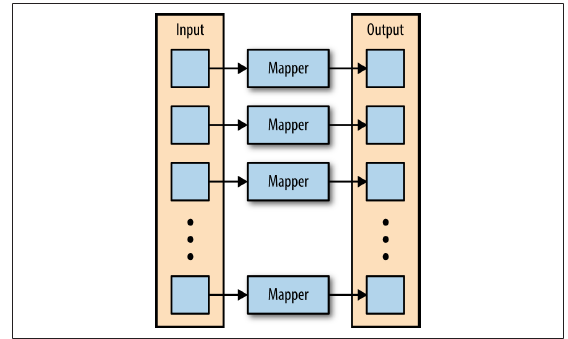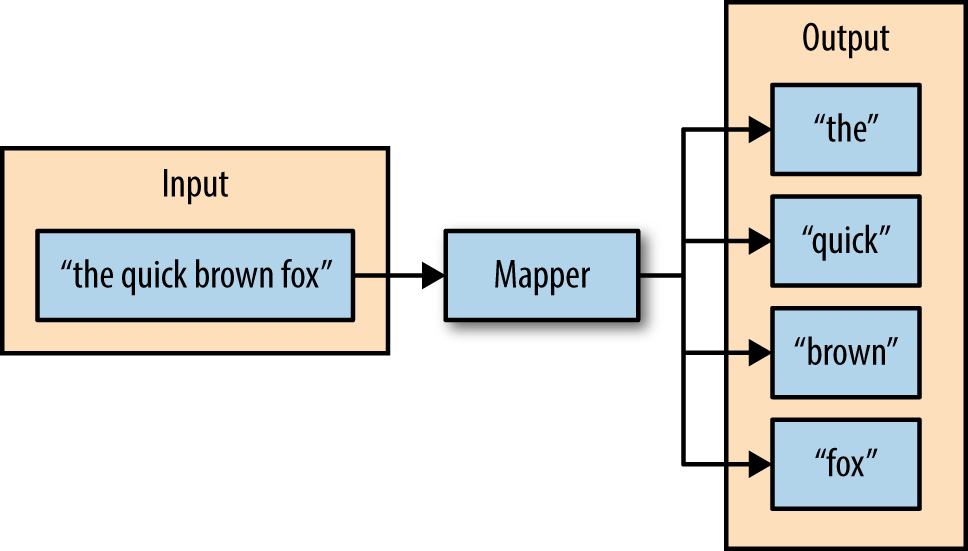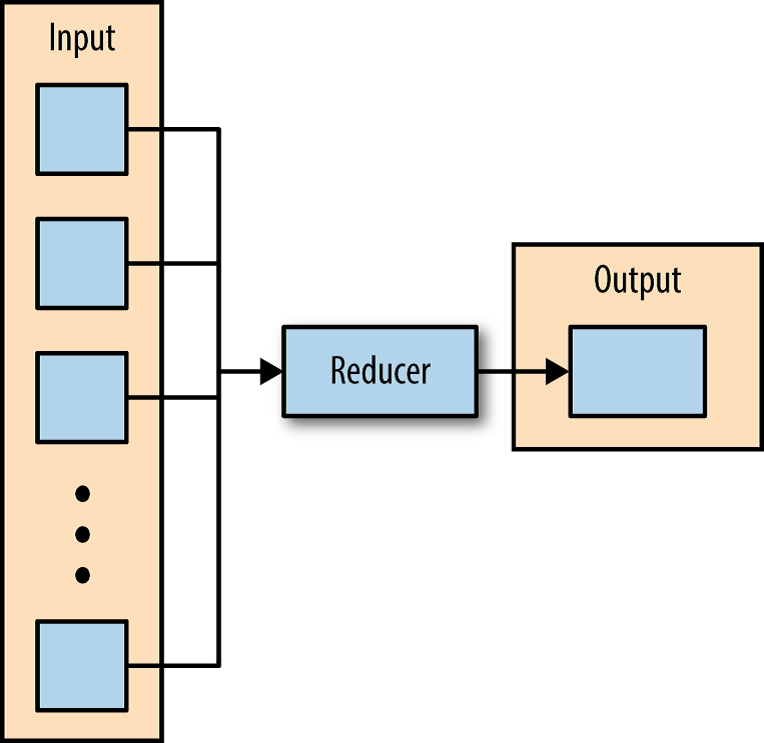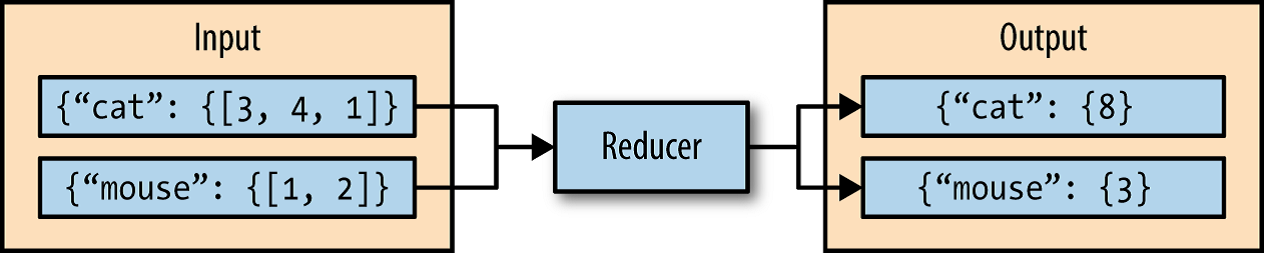# MapReduce with Python

## An introduction to the MapReduce programming model and understanding how data flows via the different stages of the model.### Introduction

MapReduce with Python is a programming model. It allows big volumes of data to be processed and created by dividing work into independent tasks. It further enables performing the tasks in parallel across a cluster of machines.

The MapReduce programming style was stirred by the functional programming constructs map and reduce. Those are usually used to process lists of data. Each Map‐Reduce program converts a list of input data elements into a list of output data elements twice at a high level. That is transformed once in the map phase and once in the reduce phase.

In this article, we will introduce the MapReduce programming model. Also, describe how data flows via the different stages of the model.

### Description

The MapReduce structure is composed of three major phases.

• Map

• Shuffle and sort

• Reduce

### Map

• The first stage of a MapReduce application is the map stage.

• A function that is called the mapper, routes a series of key-value pairs inside the map stage.

• The mapper serially processes every key-value pair separately, creating zero or more output key-value pairs.

• See the below figure in which the mapper is applied to every input key-value pair, creating an output key-value pair• Consider a mapper whose drive is to change sentences into words for instance.

• The input to this mapper will be strings that comprise sentences.

• The mapper’s function will be to divide the sentences into words and output the words.

• See the below figure in which the input of the mapper is a string, and the function of the mapper is to divide the input into spaces.

• The subsequent output is the individual words from the mapper’s input.### Shuffle and Sort

• The second stage of MapReduce is the shuffle and sort.

• The intermediate outputs from the map stage are moved to the reducers as the mappers bring into being completing.

• This process of moving output from the mappers to the reducers is recognized as shuffling.

• Shuffling is moved by a divider function, named the partitioner.

• The partitioner is used to handle the flow of key-value pairs from mappers to reducers.

• The partitioner is provided the mapper’s output key and the number of reducers.

• It returns the index of the planned reducer.

• The partitioner makes sure that all of the values for the same key are sent to the matching reducer.

• The default partitioner is identified as hash-based.

• It calculates a hash value of the mapper’s output key and allocates a partition based on this result.

• The last stage before the reducers begin processing data is the sorting process.

• The intermediate keys and values for every partition are organized by the Hadoop framework before being offered to the reducer.

### Reduce

• The third stage of MapReduce is the reduce stage.

• An iterator of values is given to a function known as the reducer inside the reducer phase.

• The iterator of values is a non-single set of values for every unique key from the output of the map stage.

• The reducer sums the values for every single key and yields zero or more output key-value pairs.

• See the below figure in which the reducer iterates over the input values, creating an output key-value pair.• Consider a reducer whose drive is to sum all of the values for a key as an instance.

• The input to this reducer is an iterator of altogether of the values for a key.

• The reducer calculates all of the values.

• The reducer then outputs a key-value pair that comprises the input key and the sum of the input key values.

• See the below figure in which the reducer sums the values for the keys “cat” and “mouse”.### A Python Example

• The WordCount application may be applied to prove how the Hadoop streaming utility may run Python as a MapReduce application on a Hadoop cluster.

• Following are two Python programs.

• py and reducer.py.

• py is the Python program that applies the logic in the map stage of WordCount.

• It states data from stdin, splits the lines into words.

• It outputs every word with its intermediate count to stdout.

• The code in the below example implements the logic in mapper.py.

#!/usr/bin/env python import sys # Read each line from stdin **for **line **in **sys.stdin: # Get the words in each line words = line.split() # Generate the count for each word **for **word **in **words: # Write the key-value pair to stdout to be processed by # the reducer. # The key is anything before the first tab character and the #value is anything after the first tab character. print '{0}\t{1}'.format(word, 1)

• py is the Python program that applies the logic in the reduce stage of WordCount.

• It delivers the results of mapper.py from stdin, sums the rates of each word, and writes the result to stdout.

• The code in the below example applies the logic in reducer.py.

#!/usr/bin/env python import sys curr*word = None curr_count = 0 # Process each key-value pair from the mapper **for **line in sys.stdin: # Get the key and value from the current line word, count = line.split('\t') # Convert the count to an int count = int(count) # If the current word is the same as the previous word,_ # increment its count, otherwise print the words count # to stdout **if **word == currword: curr_count += count else: # Write word and its number of occurrences as a key-value_ # pair to stdout **if **currword: print '{0}\t{1}'.format(curr_word, curr_count) curr_word = word curr_count = count *# Output the count for the last word_ **if **curr_word == word: print '{0}\t{1}'.format(curr_word, curr_count)

• When the mapper and reducer programs are performing well against tests, they may be run as a MapReduce application using the Hadoop streaming utility.

• Below is the command to run the Python programs mapper.py and reducer.py on a Hadoop cluster.